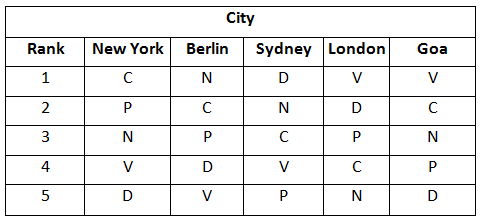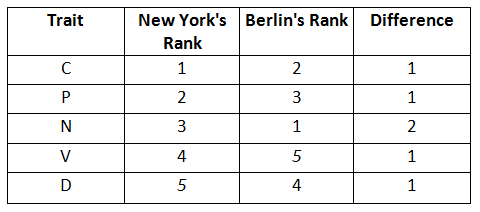### Reasoning for RBI Grade A: Practice exercises for Reasoning for RBI Grade A

Directions for the question set:
Answer the questions on the basis of the information given below.
A study was conducted to ascertain the relative importance that employees in five different cities assigned to five different traits in their Chief Executive Officers. The traits were compassion (C), decisiveness (D), negotiation skills (N), public visibility (P), and Vision (V). The level of dissimilarity between two cities is the maximum difference in the ranks allotted by the two cities to any of the five traits. The following table indicates the rank order of the five traits for each city.Question 1: Which amongst the following cities is most dissimilar to New York?
(a) Berlin
(b) Sydney
(c) London
(d) Goa

Question 2: Which of the following pairs of cities are most dissimilar?
(a) Berlin & Sydney
(b) New York & Berlin
(c) London & Sydney
(d) Goa & Sydney

Question 3: Three of the following four pairs of cities have identical levels of dissimilarity. Which pair is the odd one out ?
(a) London & Berlin
(b) Berlin & Goa
(c) Goa & Sydney
(d) Sydney & London

Question 4: Which of the following cities is least dissimilar to New York ?
(a) Berlin
(b) Sydney
(c) London
(d) Goa

### Answers and Explanations: Click the down arrow to expand

Answers 1: (b) Dissimilarity is the maximum difference between the ranks allotted by the city’s population to any particular category. For example, London and New York have a dissimilarity of (4-1=3) in the ‘C’ category. Another example, dissimilarity between New York and Berlin:From the above table the maximum difference is 2. ∴ The dissimilarity level between New York and Berlin is 2.

Hence from the similar calculations, the highest level of dissimilarity is 4 which is for Sydney and New York in ‘D’ category. The correct option is (b).

Answers 2: (d) Checking each option pair one by one The level of dissimilarity between Berlin and Sydney is for the parameter D (4 –1) = 3 New York and Berlin is for the parameter N (3 –1) = 2 London and Sydney is for the parameter V (4 – 1) = 3 Goa and Sydney is for the parameter D (5 –1) = 4 The correct option is (d).

Answers 3: (d) The level of dissimilarity between London and Berlin is for the parameter V (5 – 1) = 4 Berlin and Goa is for the parameter V (5 –1) = 4 Goa and Sydney is for the parameter D (5 –1) = 4 Sydney and London is for the parameter V (4 – 1) = 3

Hence the last option is the odd one out. The correct option is (d).

Answers 4: (a) Calculating the level of dissimilarity for New York with remaining cities Berlin : For the parameter N (3 –1) = 2 Sydney : For the parameter D (5 –1) = 4 London : For the parameter C (4 –1) = 3 Goa : For the parameter V (4 –1) = 3 ∴ The least level of dissimilarity is 2 which is for Berlin. The correct option is (a).

Extra tips for Reasoning for RBI Grade A exam:
• It is very important to develop a strategy or an attempt plan that you should abide by in Reasoning for RBI Grade A exam.
• Use option elimination method wherever applicable to save time.
• Make notes while preparing for Reasoning for RBI Grade A exam and use them to revise concepts before the exam.